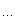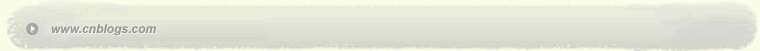Impossible is nothing 爱过知情重醉过知酒浓 　　花开花谢终是空 　　缘份不停留像春风来又走 　　女人如花花似梦

 < 2023年11月 >
2930311234
567891011
12131415161718
19202122232425
262728293012
3456789

• 随笔 - 8
• 文章 - 91
• 评论 - 16
• 引用 - 0

# 相册

•

### 评论排行榜

#include  < iostream >//  返回类型,大小分别为1、2typedef  char  RT1;typedef
struct{char  a[ 2 ];}
RT2;//  选择这个版本将返回char,大小为1template < typename T >  RT1 test(typename T::X  const * ){int     dumy     =   0 ;dumy
++ ;}//  选择这个版本将返回char,大小为2template < typename T >  RT2 test(){int     dumy     =   0 ;dumy
++ ;}//  依靠编译器自动选择test函数,然后依据返回类型判断是否含有Xtemplate < typename T >bool  type_has_member_type_X(){return  ( sizeof (test < T > ( 0 ))  ==   1 );}//  有X类型的类class  HasX{public :class  X{}
;}
;//  无X类型的类class  NonX{public :class  Y{}
;}
;int  _tmain( int  argc, _TCHAR *  argv[]){//  编译器选择重载函数策略:选择类型最匹配版本//  倾向于把0转化为常量空指针,所以选择返回为RT1版本if (type_has_member_type_X < HasX > ()){std::cout
<<   " Has X "   <<  std::endl;}else{std::cout
<<   " Non X "   <<  std::endl;}//  没有T::X,所以选择返回为RT2版本if (type_has_member_type_X < NonX > ()){std::cout
<<   " Has X "   <<  std::endl;}else{std::cout
<<   " Non X "   <<  std::endl;}return   0 ;}

Has X
Non X
posted on 2006-04-06 00:17 笑笑生 阅读(269) 评论(0)  编辑 收藏 引用 所属分类: C++语言Copyright © 笑笑生 Powered by: 博客园 模板提供：沪江博客